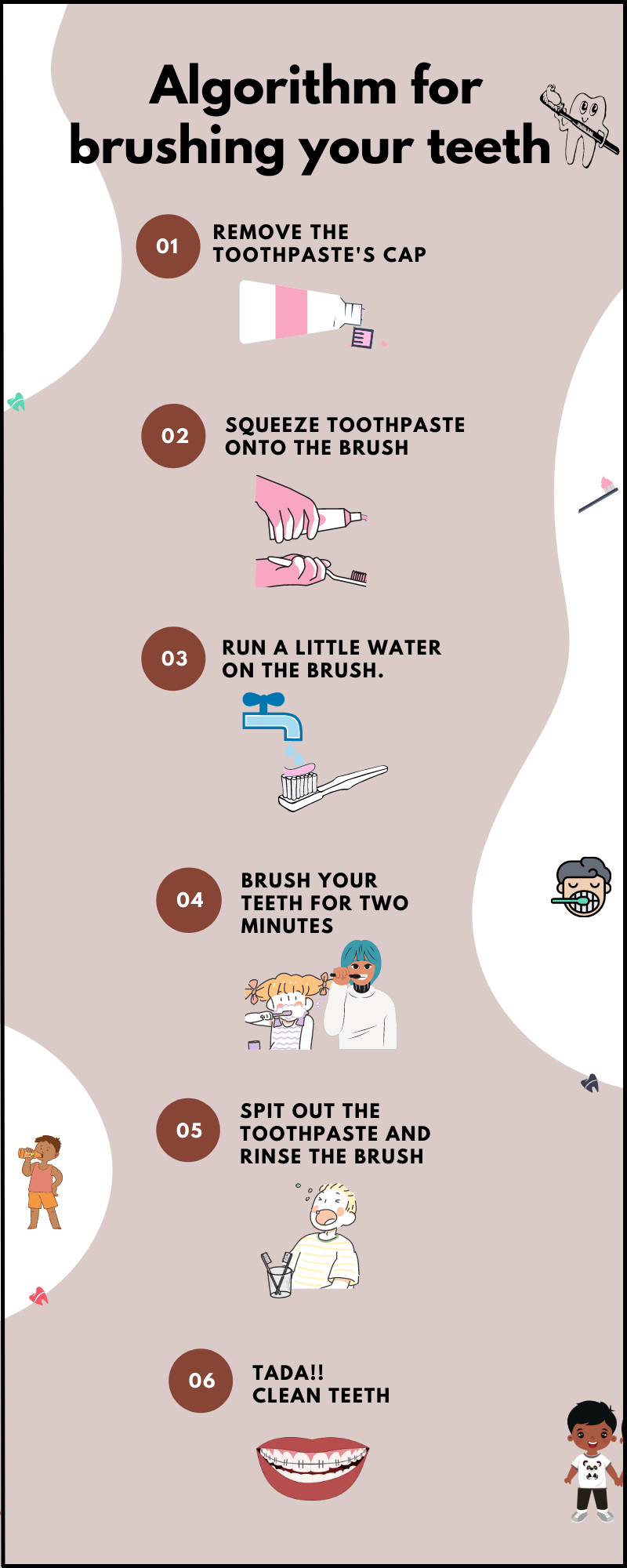Courses
Courses for Kids
Free study material
Free LIVE classes
More

# Algorithm - A Set of Rules## Introduction to Algorithms

Describing algorithms in simple words is not as difficult as we thought it is going to be. An algorithm can be defined as a set of instructions in computer science, programming, and Mathematics with the primary goal of solving a problem, doing a specific task, or performing a calculation. An algorithm is, in some ways, a very particular specification for processing data, doing calculations, and a number of other tasks.

A nice way to explain an algorithm is to state that whoever builds it is the one who determines the game's rules. Actually, algorithms are the things that define how things work and react to our actions. So, the person or machine who creates them is creating all of the possible reactions that will occur when we do according to their instructions. In this article, we're going to look at a word that you might already be familiar with. That word is Algorithm. We're also going to discuss some examples of algorithms.

## What is an Algorithm?

It's a set of step-by-step instructions placed in the correct order to do something useful. Algorithms are used to programme all of the devices we use. Everything from computers and tablets to video games consoles and smartphones to your home's microwave oven or washing machine, as well as larger things such as cars, robots, and even space rockets, rely on the algorithms written into them by humans.

An algorithm is anything that involves doing a series of tasks one after the other.

Let's assume you're brushing your teeth. Consider that for a moment. You remove the toothpaste cap. Squeeze a tiny portion of toothpaste onto the brush. Apply a small amount of water to the brush. Then keep it in your mouth. Brush your teeth for two minutes in different parts of your mouth, then spit out the toothpaste and rinse the brush.Algorithm

That's an algorithm!

Cooking delicious food, checking the timetable, playing a board game, performing a dance, and doing a Maths calculation are all examples of algorithms that we use on a regular basis.

## Algorithm in Maths

In Mathematics, an algorithm is a procedure that describes a sequence of steps that can be used to solve a mathematical problem. In many fields, algorithms are used, but the most common example is a step-by-step procedure used in long divisions. Let’s observe this Mathematical problem, “What is 69 divided by 3?”

The answer can be achieved by the following algorithm below:

• How many times does 3 go into 6?

• How many are left over? 0

• Now take 9.

• How many times does 3 go into 9?

• The answer is 3 with a remainder of zero.

• And of course, the answer is 23 with a remainder of 0.

## Fun Fact

A Mathematical algorithm is quite similar to a computer science or app development algorithm. Both have the same meaning, definition, and description.

## Conclusion

In subjects like Maths and science, algorithmic thinking, or the ability to identify the main steps to solve a problem, is important. Algorithms are used by children without their knowledge all of the time, especially in Maths. In order to answer a long division problem, students use a taught algorithm to repeat through the digits of the number they're dividing. The kid must divide, multiply, and subtract each digit of the dividend. Algorithmic thinking helps kids to analyse problems and formulate solutions in terms of specific steps in a procedure.

Last updated date: 18th Sep 2023
Total views: 119.4k
Views today: 1.19k

## FAQs on Algorithm - A Set of Rules

1. How to teach algorithms to kids?

Algorithms are a critical part of any Mathematical curriculum. Traditional strategies include rote memorization of ancient algorithms. However, over time, modern teachers have begun to develop curricula to effectively teach the concept of algorithms, which is that there are multiple ways to solve complex problems by breaking them down into a series of procedural steps. Developing algorithmic thinking is the process of allowing a kid to find new approaches to solve problems.

2. What is a simple real-life example to describe an algorithm?

Step-by-step instructions for making a smoothie are as follows:

• Add fruits to the blender.

• Pour milk into the blender.

• Put on the lid of the blender.

• Switch on the blender.

Consider what would happen if one of the steps was skipped or the order was reversed. It's likely that we'll turn on the blender with nothing in it. Or there could be no fruit in the blender and only milk. So, the procedure is very important, which is nothing but an example of the algorithm.